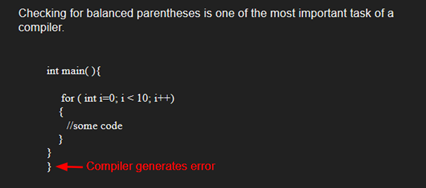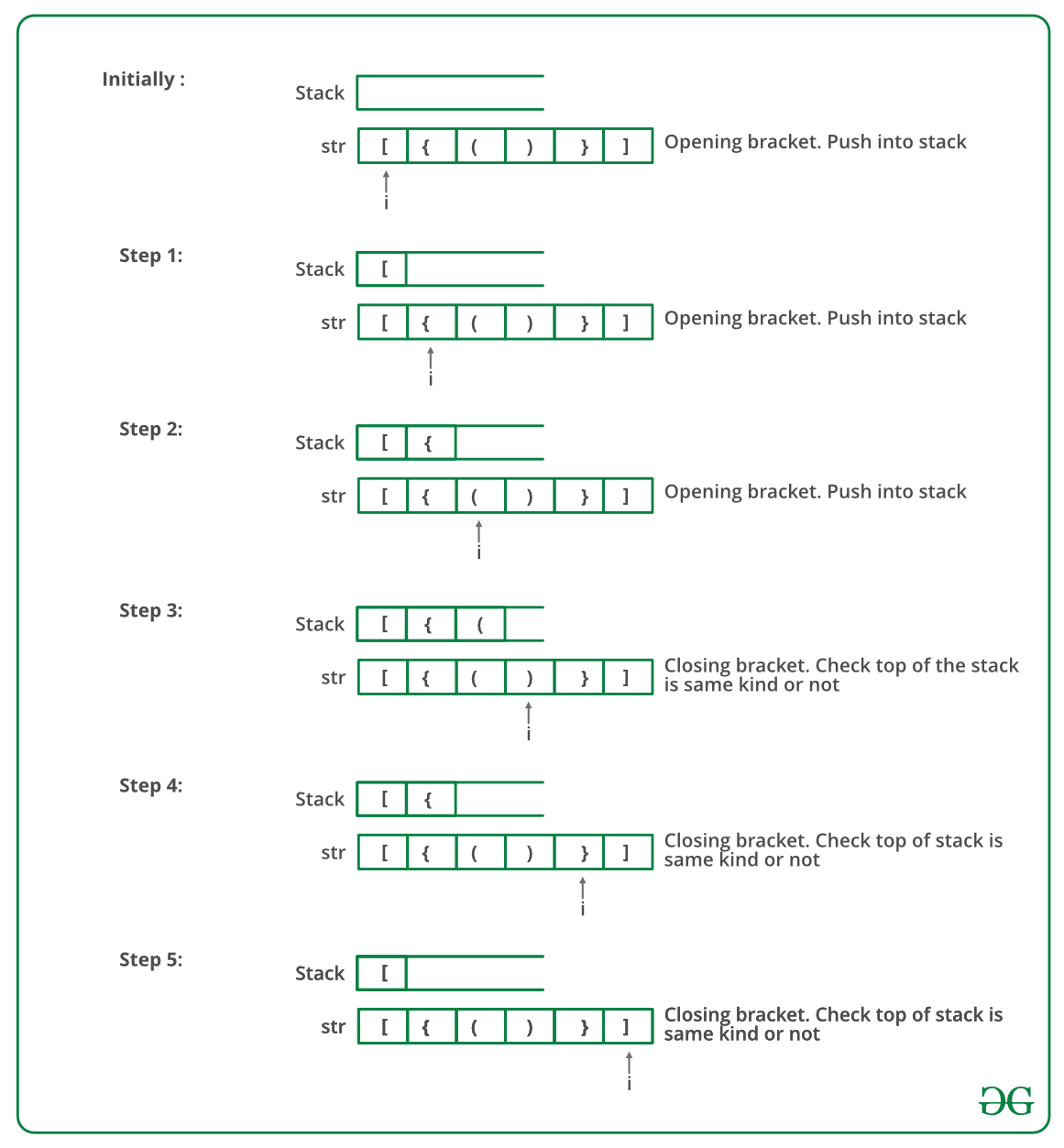# Check for Balanced Brackets in an expression (well-formedness) using Stack

• Difficulty Level : Easy
• Last Updated : 26 Apr, 2022

Given an expression string exp, write a program to examine whether the pairs and the orders of “{“, “}”, “(“, “)”, “[“, “]” are correct in exp.

Example

Input: exp = “[()]{}{[()()]()}”
Output: Balanced

Input: exp = “[(])”
Output: Not BalancedAlgorithm:

• Declare a character stack S.
• Now traverse the expression string exp.
1. If the current character is a starting bracket (‘(‘ or ‘{‘ or ‘[‘) then push it to stack.
2. If the current character is a closing bracket (‘)’ or ‘}’ or ‘]’) then pop from stack and if the popped character is the matching starting bracket then fine else brackets are not balanced.
• After complete traversal, if there is some starting bracket left in stack then “not balanced”

Below image is a dry run of the above approach:Below is the implementation of the above approach:

## C++

 `// CPP program to check for balanced brackets.``#include ``using` `namespace` `std;` `// function to check if brackets are balanced``bool` `areBracketsBalanced(string expr)``{ ``    ``stack<``char``> temp;``        ``for``(``int` `i=0;i

## C

 `#include ``#include ``#define bool int` `// structure of a stack node``struct` `sNode {``    ``char` `data;``    ``struct` `sNode* next;``};` `// Function to push an item to stack``void` `push(``struct` `sNode** top_ref, ``int` `new_data);` `// Function to pop an item from stack``int` `pop(``struct` `sNode** top_ref);` `// Returns 1 if character1 and character2 are matching left``// and right Brackets``bool` `isMatchingPair(``char` `character1, ``char` `character2)``{``    ``if` `(character1 == ``'('` `&& character2 == ``')'``)``        ``return` `1;``    ``else` `if` `(character1 == ``'{'` `&& character2 == ``'}'``)``        ``return` `1;``    ``else` `if` `(character1 == ``'['` `&& character2 == ``']'``)``        ``return` `1;``    ``else``        ``return` `0;``}` `// Return 1 if expression has balanced Brackets``bool` `areBracketsBalanced(``char` `exp``[])``{``    ``int` `i = 0;` `    ``// Declare an empty character stack``    ``struct` `sNode* stack = NULL;` `    ``// Traverse the given expression to check matching``    ``// brackets``    ``while` `(``exp``[i])``    ``{``        ``// If the exp[i] is a starting bracket then push``        ``// it``        ``if` `(``exp``[i] == ``'{'` `|| ``exp``[i] == ``'('` `|| ``exp``[i] == ``'['``)``            ``push(&stack, ``exp``[i]);` `        ``// If exp[i] is an ending bracket then pop from``        ``// stack and check if the popped bracket is a``        ``// matching pair*/``        ``if` `(``exp``[i] == ``'}'` `|| ``exp``[i] == ``')'``            ``|| ``exp``[i] == ``']'``) {` `            ``// If we see an ending bracket without a pair``            ``// then return false``            ``if` `(stack == NULL)``                ``return` `0;` `            ``// Pop the top element from stack, if it is not``            ``// a pair bracket of character then there is a``            ``// mismatch.``            ``// his happens for expressions like {(})``            ``else` `if` `(!isMatchingPair(pop(&stack), ``exp``[i]))``                ``return` `0;``        ``}``        ``i++;``    ``}` `    ``// If there is something left in expression then there``    ``// is a starting bracket without a closing``    ``// bracket``    ``if` `(stack == NULL)``        ``return` `1; ``// balanced``    ``else``        ``return` `0; ``// not balanced``}` `// Driver code``int` `main()``{``    ``char` `exp`` = ``"{()}[]"``;` `    ``// Function call``    ``if` `(areBracketsBalanced(``exp``))``        ``printf``(``"Balanced \n"``);``    ``else``        ``printf``(``"Not Balanced \n"``);``    ``return` `0;``}` `// Function to push an item to stack``void` `push(``struct` `sNode** top_ref, ``int` `new_data)``{``    ``// allocate node``    ``struct` `sNode* new_node``        ``= (``struct` `sNode*)``malloc``(``sizeof``(``struct` `sNode));` `    ``if` `(new_node == NULL) {``        ``printf``(``"Stack overflow n"``);``        ``getchar``();``        ``exit``(0);``    ``}` `    ``// put in the data``    ``new_node->data = new_data;` `    ``// link the old list off the new node``    ``new_node->next = (*top_ref);` `    ``// move the head to point to the new node``    ``(*top_ref) = new_node;``}` `// Function to pop an item from stack``int` `pop(``struct` `sNode** top_ref)``{``    ``char` `res;``    ``struct` `sNode* top;` `    ``// If stack is empty then error``    ``if` `(*top_ref == NULL) {``        ``printf``(``"Stack overflow n"``);``        ``getchar``();``        ``exit``(0);``    ``}``    ``else` `{``        ``top = *top_ref;``        ``res = top->data;``        ``*top_ref = top->next;``        ``free``(top);``        ``return` `res;``    ``}``}`

## Java

 `// Java program for checking``// balanced brackets``import` `java.util.*;` `public` `class` `BalancedBrackets {` `    ``// function to check if brackets are balanced``    ``static` `boolean` `areBracketsBalanced(String expr)``    ``{``        ``// Using ArrayDeque is faster than using Stack class``        ``Deque stack``            ``= ``new` `ArrayDeque();` `        ``// Traversing the Expression``        ``for` `(``int` `i = ``0``; i < expr.length(); i++)``        ``{``            ``char` `x = expr.charAt(i);` `            ``if` `(x == ``'('` `|| x == ``'['` `|| x == ``'{'``)``            ``{``                ``// Push the element in the stack``                ``stack.push(x);``                ``continue``;``            ``}` `            ``// If current character is not opening``            ``// bracket, then it must be closing. So stack``            ``// cannot be empty at this point.``            ``if` `(stack.isEmpty())``                ``return` `false``;``            ``char` `check;``            ``switch` `(x) {``            ``case` `')'``:``                ``check = stack.pop();``                ``if` `(check == ``'{'` `|| check == ``'['``)``                    ``return` `false``;``                ``break``;` `            ``case` `'}'``:``                ``check = stack.pop();``                ``if` `(check == ``'('` `|| check == ``'['``)``                    ``return` `false``;``                ``break``;` `            ``case` `']'``:``                ``check = stack.pop();``                ``if` `(check == ``'('` `|| check == ``'{'``)``                    ``return` `false``;``                ``break``;``            ``}``        ``}` `        ``// Check Empty Stack``        ``return` `(stack.isEmpty());``    ``}` `    ``// Driver code``    ``public` `static` `void` `main(String[] args)``    ``{``        ``String expr = ``"([{}])"``;` `        ``// Function call``        ``if` `(areBracketsBalanced(expr))``            ``System.out.println(``"Balanced "``);``        ``else``            ``System.out.println(``"Not Balanced "``);``    ``}``}`

## Python3

 `# Python3 program to check for``# balanced brackets.` `# function to check if``# brackets are balanced`  `def` `areBracketsBalanced(expr):``    ``stack ``=` `[]` `    ``# Traversing the Expression``    ``for` `char ``in` `expr:``        ``if` `char ``in` `[``"("``, ``"{"``, ``"["``]:` `            ``# Push the element in the stack``            ``stack.append(char)``        ``else``:` `            ``# IF current character is not opening``            ``# bracket, then it must be closing.``            ``# So stack cannot be empty at this point.``            ``if` `not` `stack:``                ``return` `False``            ``current_char ``=` `stack.pop()``            ``if` `current_char ``=``=` `'('``:``                ``if` `char !``=` `")"``:``                    ``return` `False``            ``if` `current_char ``=``=` `'{'``:``                ``if` `char !``=` `"}"``:``                    ``return` `False``            ``if` `current_char ``=``=` `'['``:``                ``if` `char !``=` `"]"``:``                    ``return` `False` `    ``# Check Empty Stack``    ``if` `stack:``        ``return` `False``    ``return` `True`  `# Driver Code``if` `__name__ ``=``=` `"__main__"``:``    ``expr ``=` `"{()}[]"` `    ``# Function call``    ``if` `areBracketsBalanced(expr):``        ``print``(``"Balanced"``)``    ``else``:``        ``print``(``"Not Balanced"``)` `# This code is contributed by AnkitRai01 and improved``# by Raju Pitta`

## C#

 `// C# program for checking``// balanced Brackets``using` `System;``using` `System.Collections.Generic;` `public` `class` `BalancedBrackets {``    ``public` `class` `stack {``        ``public` `int` `top = -1;``        ``public` `char``[] items = ``new` `char``;` `        ``public` `void` `push(``char` `x)``        ``{``            ``if` `(top == 99)``            ``{``                ``Console.WriteLine(``"Stack full"``);``            ``}``            ``else` `{``                ``items[++top] = x;``            ``}``        ``}` `        ``char` `pop()``        ``{``            ``if` `(top == -1)``            ``{``                ``Console.WriteLine(``"Underflow error"``);``                ``return` `'\0'``;``            ``}``            ``else``            ``{``                ``char` `element = items[top];``                ``top--;``                ``return` `element;``            ``}``        ``}` `        ``Boolean isEmpty()``        ``{``            ``return` `(top == -1) ? ``true` `: ``false``;``        ``}``    ``}` `    ``// Returns true if character1 and character2``    ``// are matching left and right brackets */``    ``static` `Boolean isMatchingPair(``char` `character1,``                                  ``char` `character2)``    ``{``        ``if` `(character1 == ``'('` `&& character2 == ``')'``)``            ``return` `true``;``        ``else` `if` `(character1 == ``'{'` `&& character2 == ``'}'``)``            ``return` `true``;``        ``else` `if` `(character1 == ``'['` `&& character2 == ``']'``)``            ``return` `true``;``        ``else``            ``return` `false``;``    ``}` `    ``// Return true if expression has balanced``    ``// Brackets``    ``static` `Boolean areBracketsBalanced(``char``[] exp)``    ``{``        ``// Declare an empty character stack */``        ``Stack<``char``> st = ``new` `Stack<``char``>();` `        ``// Traverse the given expression to``        ``//   check matching brackets``        ``for` `(``int` `i = 0; i < exp.Length; i++)``        ``{``            ``// If the exp[i] is a starting``            ``// bracket then push it``            ``if` `(exp[i] == ``'{'` `|| exp[i] == ``'('``                ``|| exp[i] == ``'['``)``                ``st.Push(exp[i]);` `            ``//  If exp[i] is an ending bracket``            ``//  then pop from stack and check if the``            ``//   popped bracket is a matching pair``            ``if` `(exp[i] == ``'}'` `|| exp[i] == ``')'``                ``|| exp[i] == ``']'``) {` `                ``// If we see an ending bracket without``                ``//   a pair then return false``                ``if` `(st.Count == 0)``                ``{``                    ``return` `false``;``                ``}` `                ``// Pop the top element from stack, if``                ``// it is not a pair brackets of``                ``// character then there is a mismatch. This``                ``// happens for expressions like {(})``                ``else` `if` `(!isMatchingPair(st.Pop(),``                                         ``exp[i])) {``                    ``return` `false``;``                ``}``            ``}``        ``}` `        ``// If there is something left in expression``        ``// then there is a starting bracket without``        ``// a closing bracket` `        ``if` `(st.Count == 0)``            ``return` `true``; ``// balanced``        ``else``        ``{``            ``// not balanced``            ``return` `false``;``        ``}``    ``}` `    ``// Driver code``    ``public` `static` `void` `Main(String[] args)``    ``{``        ``char``[] exp = { ``'{'``, ``'('``, ``')'``, ``'}'``, ``'['``, ``']'` `};` `        ``// Function call``        ``if` `(areBracketsBalanced(exp))``            ``Console.WriteLine(``"Balanced "``);``        ``else``            ``Console.WriteLine(``"Not Balanced "``);``    ``}``}` `// This code is contributed by 29AjayKumar`

## Javascript

 ``
Output
`Balanced`

Time Complexity: O(n)
Auxiliary Space: O(n) for stack.

Please write comments if you find any bug in above codes/algorithms, or find other ways to solve the same problem

My Personal Notes arrow_drop_up